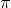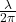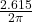A loudspeaker at the origin emits a 130 Hz tone on a day when the speed of sound is 340 m/s. The phase difference between two points on the

Question

A loudspeaker at the origin emits a 130 Hz tone on a day when the speed of sound is 340 m/s. The phase difference between two points on the x-axis is 5.6 rad.What is the distance between these two points

in progress 0
5 months 2021-08-15T04:20:55+00:00 1 Answers 12 views 0

distance between two points having phase difference 5.6 rad is

Φ = 2.33 m

Explanation:

given

frequency, f = 130Hz

speed of sound in air, v = 340m/s

distance between two crust or through with phase difference 2= λ
(wavelength)

note: distance between two points having a phase difference =∴ distance between two points having phase difference of 5.6 rad is =×5.6

Recall

v = f × λ

speed = frequency × wavelength

wavelength = speed/frequency

λ  = v/f

λ  = 340/130 = 2.615 m

∴ distance between two points having phase difference 5.6 rad is =×5.6 = 2.33 m